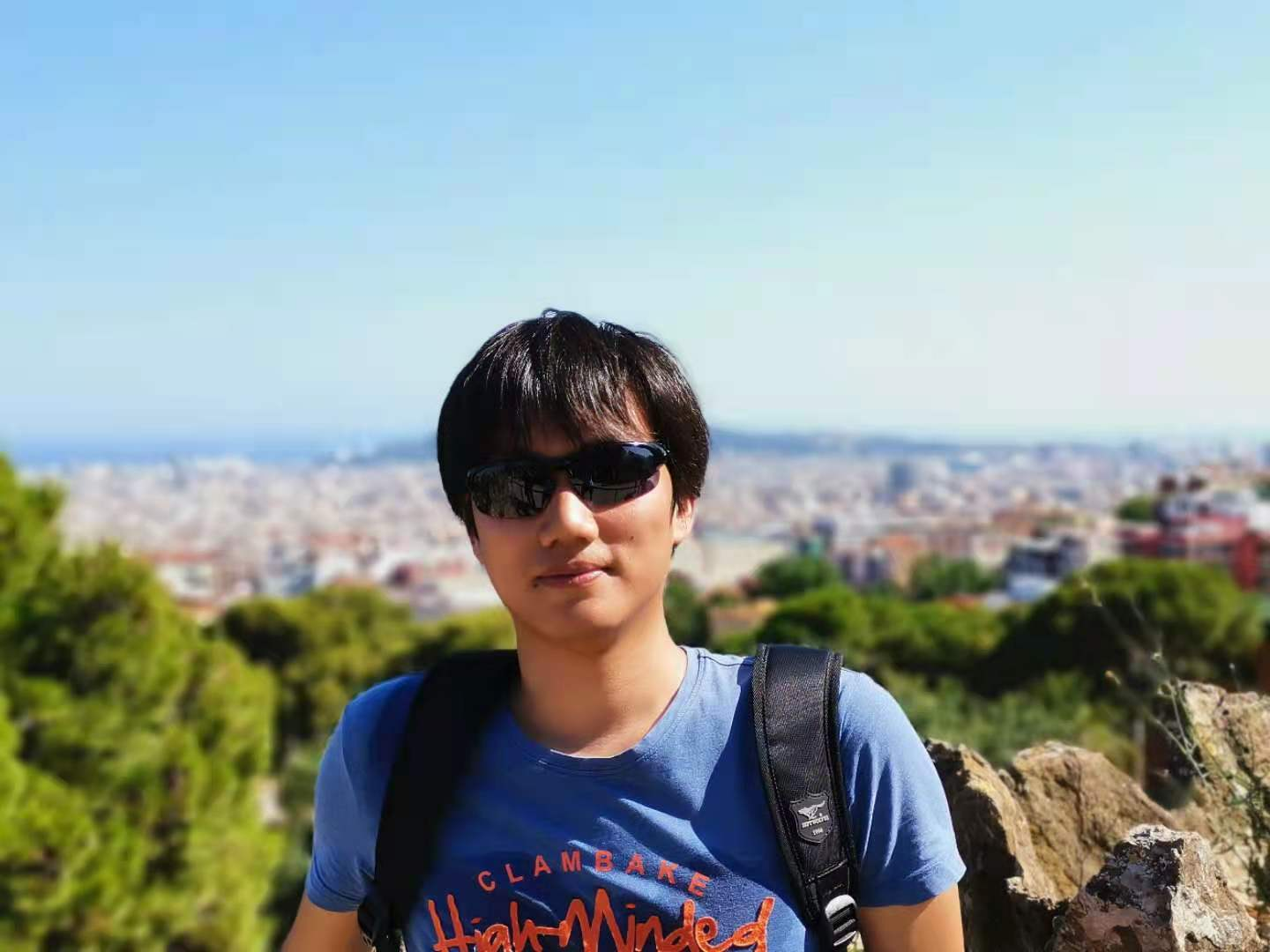# For: Learning Invariant Representations for Reinforcement Learning without Reconstruction

96

## 算法（Deep Bisimulation for Control, DBC）

### Bisimulation

\begin{align*} \mathcal{R} (s_i, a) &= \mathcal{R}\mathcal(s_j, a) & \forall a \in \mathcal{A} \tag{1}\\ \mathcal{P}(G\vert s_i, a) &= \mathcal{P}(G\vert s_j, a) & \forall a \in \mathcal{A}, \forall G \in \mathcal{S}_B \tag{2} \end{align*}

$$d(s_i, s_j) = \max_{a\in\mathcal{A}} (1-c)\cdot \vert \mathcal{R}_{s_i}^a - \mathcal{R}_{s_j}^a \vert + c \cdot W_1(\mathcal{P}_{s_i}^a, \mathcal{P}_{s_j}^a;d) \tag{3}$$

Bisimulation 指标直接反映了两个状态的行为等效程度。以图一为例，图一中三个驾驶背景（左上、右上、右下）应该是行为等效的。如果我们能够学习到一个编码器，它编码得到的状态可以直接反映Bisimulation 指标，那么此编码器的范化性应该会非常好。

## 参考文献

 Zhang, Amy, et al. "Learning invariant representations for reinforcement learning without reconstruction."arXiv preprint arXiv:2006.10742(2020).

 Wasserstein distance between two Gaussians,https://djalil.chafai.net/blog/2010/04/30/wasserstein-distance-between-two-gaussians/

 Givens, Clark R., and Rae Michael Shortt. "A class of Wasserstein metrics for probability distributions."Michigan Mathematical Journal31.2 (1984): 231-240.

 Chua, Kurtland, et al. "Deep reinforcement learning in a handful of trials using probabilistic dynamics models."Advances in neural information processing systems31 (2018).

 Haarnoja, Tuomas, et al. "Soft actor-critic: Off-policy maximum entropy deep reinforcement learning with a stochastic actor."International conference on machine learning. PMLR, 2018.

Article Tags
[本]通信工程@河海大学 & [硕]CS@清华大学

0
96
0

More RecommendationsApril 29, 2022April 13, 2022April 5, 2022March 30, 2022